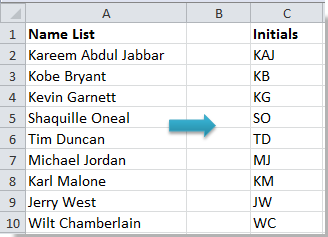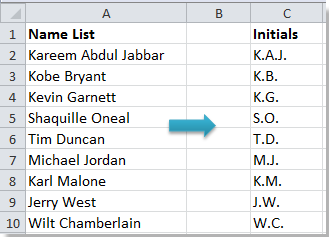## How to extract initials from names in Excel?

When you are processing customer records or doing mail merge, getting initials from a given name may be useful for you, for instance, HG for Hal Greer. So here I introduce some tricks for you to quickly extract initials from the name list in Excel.

Extract initials with formula in Excel

Extract initials use Defined Function in Excel

####Extract initials with formula in Excel###### Save 50% of your time, and reduce thousands of mouse clicks for you every day!

First method is using formula to extract initials from names in Microsoft Excel.

Select a blank cell, for instance, Cell C2, type this formula =LEFT(A2)&IF(ISNUMBER(FIND(" ",A2)),MID(A2,FIND(" ",A2)+1,1),"")&IF(ISNUMBER(FIND(" ",A2,FIND(" ",A2)+1)),MID(A2,FIND(" ",A2,FIND(" ",A2)+1)+1,1),"")(A2 is the first cell of your name list), press Enter button, then drag the fill handle to fill the range. Now you see all initials of each name are extracted from the name list.####Extract initials use Defined Function in Excel

Besides formula, you can use the Defined Function to extract initials from specified names easily in Microsoft Excel.

1. Select a cell of the column you want to select and press Alt + F11 to open the Microsoft Visual Basic for Applications window.

2. In the pop-up window, click Insert > Module, then paste the following VBA code into the module.

VBA: Extract initials from names

``````Function FirstCharacters(pWorkRng As Range) As String
'Updateby20140514
Dim arr As Variant
Dim xValue As String
Dim OutValue As String
xValue = pWorkRng.Value
arr = VBA.Split(Trim(xValue))
For i = 0 To UBound(arr)
OutValue = OutValue & VBA.Left(arr(i), 1) & "."
Next
FirstCharacters = OutValue
End Function``````

3. Save the code and close the window, select a blank cell and type this formula = FirstCharacters(A2), then press Enter button, and drag the fill handle to fill the range you want. After that, you can see the initials of each name are extracted.Tip: You can change the separators "." of the initials as you need in the above VBA.

### Best Office Productivity Tools

Supports Office/Excel 2007-2021 and 365  |  Available in 44 Languages  |  Easy to Uninstall Completely

 Popular Features: Find/Highlight/Identify Duplicates   |  Delete Blank Rows   |  Combine Columns or Cells without Losing Data   |   Round without Formula ... Super Lookup: Multiple Criteria VLookup  |   Multiple Value VLookup  |   VLookup Across Multiple Sheets   |   Fuzzy Lookup .... Advanced Drop-down List: Quickly Create Drop Down List   |  Dependent Drop Down List   |  Multi-select Drop Down List .... Column Manager: Add a Specific Number of Columns   |   Move Columns   |   Unhide Columns   |   Compare Columns to Select Same & Different Cells ... Featured Features: Grid Focus   |  Design View   |   Big Formula Bar   |  Workbook & Sheet Manager   |  Resource Library (Auto Text)   |  Date Picker   |  Combine Worksheets   |  Encrypt/Decrypt Cells   |  Send Emails by List   |  Super Filter   |   Special Filter (filter bold/italic/strikethrough...) ... Top 15 Toolsets:  12 Text Tools (Add Text, Remove Characters, ...)   |   50+ Chart Types (Gantt Chart, ...)   |   40+ Practical Formulas (Calculate age based on birthday, ...)   |   19 Insertion Tools (Insert QR Code, Insert Picture from Path, ...)   |   12 Conversion Tools (Numbers to Words, Currency Conversion, ...)   |   7 Merge & Split Tools (Advanced Combine Rows, Split Cells, ...)   |   ... and more

Kutools for Excel Boasts Over 300 Features, Ensuring That What You Need Is Just A Click Away...

Supercharge Your Excel Skills: Experience Efficiency Like Never Before with Kutools for Excel  (Full-Featured 30-Day Free Trial)60-Day Unconditional Money-Back Guarantee

#### Office Tab Brings Tabbed interface to Office, and Make Your Work Much Easier

• Enable tabbed editing and reading in Word, Excel, PowerPoint, Publisher, Access, Visio and Project.
• Open and create multiple documents in new tabs of the same window, rather than in new windows.
• Increases your productivity by 50%, and reduces hundreds of mouse clicks for you every day! (Full-Featured 30-Day Free Trial)60-Day Unconditional Money-Back Guarantee

No ratings yet. Be the first to rate!
This comment was minimized by the moderator on the site
Hello,
I need to extend this code to 4 initials, but I don’t understand how to extend it.

=LEFT(A2)&IF(ISNUMBER(FIND(" ",A2)),MID(A2,FIND(" ",A2)+1,1),"")&IF(ISNUMBER(FIND(" ",A2,FIND(" ",A2)+1)),MID(A2,FIND(" ",A2,FIND(" ",A2)+1)+1,1),"")

Please can you explain how to add another layer to this formula?
This comment was minimized by the moderator on the site
Hi, use the formula like this:
``=LEFT(A2)&IF(ISNUMBER(FIND(" ",A2)),MID(A2,FIND(" ",A2)+1,1),"")&IF(ISNUMBER(FIND(" ",A2,FIND(" ",A2)+1)),MID(A2,FIND(" ",A2,FIND(" ",A2)+1)+1,1),"")&IF(ISNUMBER(FIND(" ",A2,FIND(" ",A2)+1)),MID(A2,FIND(" ",A2,FIND(" ",A2,FIND(" ",A2)+1)+1)+1,1),"")``

About the explaination, it not few words can explan. You may know what the functions in the formula is used for what destination. If you want to learn the function, you can visit this site: https://www.extendoffice.com/excel/functions.html
This comment was minimized by the moderator on the site
Thank you so much. This worked perfectly.
This comment was minimized by the moderator on the site
If u need the prefix of Mr., Ms. or Mrs. can add it in the beginning as =LEFT(A2,SEARCH(" ",A2))&
This comment was minimized by the moderator on the site
Very good formula to get the initials from a name. But if the name contains with Mr., Ms. or Mrs. then this does not work. We have to separate put this prefix first. For that you can replace the A2 with TRIM(MID(A2,SEARCH(" ",A2)+1,100)) . So the formula will be as under
=LEFT(TRIM(MID(A2,SEARCH(" ",A2)+1,100)) )&IF(ISNUMBER(FIND(" ",TRIM(MID(A2,SEARCH(" ",A2)+1,100)) )),MID(TRIM(MID(A2,SEARCH(" ",A2)+1,100)),FIND(" ",TRIM(MID(A2,SEARCH(" ",A2)+1,100)) )+1,1),"")&IF(ISNUMBER(FIND(" ",TRIM(MID(A2,SEARCH(" ",A2)+1,100)),FIND(" ",TRIM(MID(A2,SEARCH(" ",A2)+1,100)) )+1)),MID(TRIM(MID(A2,SEARCH(" ",A2)+1,100)),FIND(" ",TRIM(MID(A2,SEARCH(" ",A2)+1,100)),FIND(" ",TRIM(MID(A2,SEARCH(" ",A2)+1,100)) )+1)+1,1),"")
This comment was minimized by the moderator on the site
Thank you for this!! I had been struggling with getting initials from a last name with a space or hyphen and was able to use this formula as-is, with the addition of another IF that searched for a hyphen. A huge relief!!
This comment was minimized by the moderator on the site
I used the formula above, but if the person does not put a space between their first / last name, how do you keep that from error out?
This comment was minimized by the moderator on the site
Amazing Thank you!
This comment was minimized by the moderator on the site
hi there
I Want to sort full name in to a new format if excel cell has text character more than 25 how to show it in initials and last name
Ex 01:- name has more than 25 characters
Full Name : Mildred Dresselhaus Lene Vestergaard Hau
Show as : M.D.L Vestergaard Hau
(it may add if more initials are ther for a long name)
Ex 02:-
Full Name : Enrico Fermi
Show as : Enrico Fermi
This comment was minimized by the moderator on the site
If the text contains more than 03 words
This comment was minimized by the moderator on the site
This works!!! Thank you!
This comment was minimized by the moderator on the site
Thank you! :lol: quite useful!
This comment was minimized by the moderator on the site
lovely - thanks for this!!
There are no comments posted here yet